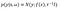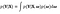# A Primer for Bayesian Deep Learning - Part 1

In modern practice, neural networks and non-parametric methods such as Gaussian processes with millions of parameters are optimised to fit datasets. It’s an open question on the generalization of such large models but it is evident that such models are very expensive to train. BDL could offer a solution to the scaling challenges of neural networks with evidence showing robustness to overfitting, uncertainty estimates, and they could easily learn from limited dataset. We can view classical training as performing approximate Bayesian inference, using the approximate posterior.

Bayesian deep learning in a more general term encompasses the intersection between probabilistic Bayesian methods and the popular deep learning. In order to fully understand BDL, a brief literature for deep learning and Bayesian modelling is needed.

# 1. Bayesian Modelling

Deep learning describes a class of machine learning models that processes information in hierarchical architectures i.e., multi-layered artificial neural networks by learning data representations . Recent successes in deep learning have propelled it in the intersections of several research areas, including computer vision, speech recognition, graphical modelling, optimization, social network filtering, pattern recognition, bioinformatics, machine translation, drug design, and signal processing, etc.

The popularity of deep learning can be attributed to the recent advances in machine learning methods, increased processing abilities like invention of powerful GPUs and the significantly lower cost of computing hardware. Over the past several years, several families of deep learning techniques have been extensively studied, e.g., Deep Belief Network (DBN), Boltzmann Machines (BM), Restricted Boltzmann Machines (RBM), Deep Boltzmann Machine (DBM), Deep Neural Networks (DNN), etc. Among various techniques, the deep convolutional neural networks, which is a discriminative deep architecture and belongs to the DNN category, has found state-of-the-art performance on various tasks and competitions in computer vision and image recognition.

In particular, the deep convolutional neural networks, which belongs to the DNN category, has found state-of-the-art performance on image classification and computer vision tasks. Specifically, the deep convolutional neural network (CNN) model consists of several convolutional layers and pooling layers, which are stacked up with one on top of another. The convolutional layer shares many weights, and the pooling layer sub-samples the output and reduces the data rate from the layer below. The weight sharing together with appropriately chosen pooling schemes, endows the CNN with some invariance properties (e.g., translation invariance).

Lately, Deep Convolutional Neural Networks (CNN) has gained popularity in performing computer vision and machine learning tasks owing to the outstanding performance achieved in performing such tasks. Yann LeCun  adopted a convolutional neural network trained globally using gradient-based methods for digit recognition. Hinton proposed the deep convolutional neural networks  that achieved first position in image classification task of ImageNet classification. Trained on more than one million images, the CNN achieved a winning top-5 test error rate of 15.3% over 1,000 classes. Recent research in CNN models has gotten better results. In , the top-5 test error rate decreased to 13.24%. This was done by concurrently training the model to classify, locate and detect objects. Consecutively, object detection has improved with advances in CNNs as documented in here.

# 2. Bayesian Modelling

Bayesian modelling defines how to do inference about hypotheses (uncertain quantities) from data (measured quantities). Learning and prediction can be seen as forms of inference. For example, suppose given observed inputs X = {x1, . . . , xN} and their corresponding outputs Y = {y1, . . . , yN}, we would like to capture the stochastic process believed to have generated our outputs i.e. parameters ω of a function .

Using the Bayesian modelling, we add some prior distribution over the space of parameters, p(ω). The prior distribution specifies which initial parameters that are likely to have produced our data before any observation. After observation, the distribution nets the more likely and less likely parameters given the observed data. For regression, we often define a likelihood distribution p(y|x,ω) — the Gaussian by which the inputs generate the outputs given some parameter setting ω.

For classification, we squash the model uncertainty to a softmax likelihood,

or a Gaussian likelihood for regression:Eq. (2.1)

with model precision τ. This can distort the model output with observation noise with variance τ−1.

Given a dataset X, Y, we then look for the posterior distribution over the space of parameters:

Consequently, we can define the posterior distribution as:

We can use the distribution to predict an output for a new input point x∗ by integrating

An important aspect of posterior evaluation is model evidence (the normaliser).Eq. (2.2)

The integration done at the Eq. (2.2) is referred to as marginalising over ω. This is the origin of the other name for the model evidence — marginal likelihood. Marginalisation for models such as Bayesian linear regression can be performed analytically and with such models, the likelihood is conjugate to the prior, and the integral can be solved with calculus.

Marginalisation is fundamental to Bayesian modelling. Therefore, preferably you want to marginalise over all uncertain quantities — i.e. average with respect to all possible models ω, each weighted by its plausibility p(ω).

But with certain interesting models, performing this marginalisation analytically becomes intractable. With such scenarios, an approximation is required.

A Primer for Bayesian Deep Learning — Part 2

# References

1. J. Dean, C. G, R. Monga, C. K, M. Devin, Q. V. Le, M. Z. Mao, M. Ranzato and P. A. T. K. Y. a. A. Y. N. A. W. Senior, “Large scale distributed deep networks,” NIPS, p. 1232–1240, 2012.
2. Y. LeCun, L. Bottou, Y. Bengio and H. P, “Gradient-based learning applied to document recognition,” Proceedings of the IEEE, vol. 86, no. 11, p. 2278–2324, 1998.
3. A. Krizhevsky, I. Sutskever and G. E. Hinton, “Imagenet classification with deep convolutional neural networks,” Advances in neural information processing systems, p. 1097–1105, 2012.
4. Pierre Sermanet, David Eigen, Xiang Zhang, Michael Mathieu, Rob Fergus, Yann LeCun, “Overfeat: Integrated recognition, localization and,” CoRR, vol. abs/1312.6229, 2013.
5. Ross Girshick, Jeff Donahue, Trevor Darrell, Jitendra Malik, “Rich feature hierarchies for accurate object detection and semantic,” CoRR, abs, vol. 1311, no. 2524, 2013.

Machine Learning Researcher- Deep Learning, Generative Models, Reinforcement Learning, Bayesian Methods, NLP, Computer Vision

## More from Robin Jackson

Machine Learning Researcher- Deep Learning, Generative Models, Reinforcement Learning, Bayesian Methods, NLP, Computer Vision